1. Projective or Homogeneous Coordinates

Here I work in the standard model of projective plane P2, consisting of the classes X= [x] = [x1, x2, x3]
of points of R3 modulo non-zero multiplicative constants. A system of projective
or homogeneous coordinates   results from a projective base (see ProjectiveBase.html ), which
is a system of four points of the plane {A, B, C, D} represented by corresponding vectors {[a], [b], [c], [d]}
of R3, such that:
(i) {a,b,c} build a basis of R3 and.
(ii)  d = a + b + c.
The projective base defines a projective coordinate system in
which we write X = u[a] + v[b] + w[c], {u,v,w} being defined modulo a non-zero
multiplicative constant, and resulting from the corresponding representetion of [x] = X, through
the basis vectors: x = ua + vb + wc.
We write simply X = uA + vB + wC (meaning a weighted sum of points of the projective plane).
{u,v,w} are the  projective coordinates of point X with respect to the system {A,B,C,D}.
In particular, points {A,B,C,D} have correspondingly the coordinates {(1,0,0), (0,1,0), (0,0,1), (1,1,1)}.
{A,B,C} are often called the basis points of the projective coordinate system.
D is often called the unit point or coordinator of the  system.2. Examples of homogeneous systems

Every triangle ABC of the (euclidean) plane together with a point D not lying on the side-lines
of the triangle defines a homogeneous system of coordinates. The mechanism to do this is
simply described by representing the triangle through coordinates in space R3 putting it into
the plane z=1. Thus, the vertices of the triangle and D being represented through the lines
(= points of P2):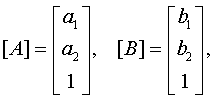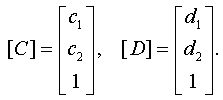The first three column vectors are those used in the definition. The fourth is determined by solving
the system: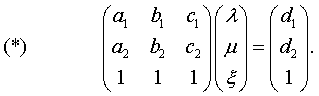This system admits a unique solution (by the non-collinearity assumption) and determines
the vectors necessary to find the homogeneous coordinates according to the definition.
In fact, the vectors to be used are: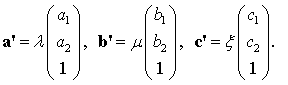To find the homogeneous coordinates in this system for a point X represented by a non-zero vector
X=[x], write the vector as a linear combination:

(0)                                                             x = ua'+vb'+wc'.

The triple (u,v,w) gives the homogeneous coordinates of X=[x] for this system of coordinates
defined by the triangle ABC and the coordinator (or unit) point D. Point D has the coordinates (1,1,1)
as required.

There are two particular systems of projective coordinates worth mentioning for their use in the
geometry of the triangle: (i) the barycentric homogeneous coordinates (see BarycentricCoordinates.html ),
and (ii) the trilinear homogeneous coordinates (see Trilinears.html ). The barycentric system for the
triangle of reference ABC results by adopting for unit the centroid G = (1/3)(A+B+C) of the triangle.
The trilinear or isogonal system of homogeneous coordinates is defined by adopting for unit
the incenter I of the triangle of reference.
The barycentric system is in some sense universal and simpler than the others since in this case the
coefficients in (*) are equal and can be taken 1. In addition to the resulting simplification, the barycenter
is an affine invariant.
Note also that in (*) used to define the system through the unit  D = (d1, d2, 1)t selected, the unknown
coefficients: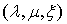are the barycentric coordinates of the point D (see BarycentricsFormulas.html ). Thus, for the trilinear
system of homogeneous coordinates this triple is (a,b,c), where the numbers are the lengths of the corresponding
sides of the triangle of reference.3. Relation to cartesian coordinates

Identifying x with a column vector xt = (x1,x2,x3) (xt denoting matrix transposition) we find the relation of the
cartesian (some times referred to as natural homogeneous coordinates of the projective plane) to projective
homogeneous coordinates using the basic matrix defined by the vertices of the triangle and equation (0).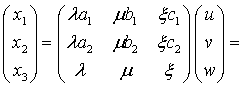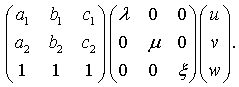It is interesting here to notice that ordinary points corresponding to cartesian coordinates
with x3=1 have homogeneous coordinates satisfying the equation: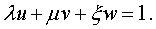Points at infinity corresponding to cartesian coordinates with x3=0 have homogeneous coordinates
satisfying the equation: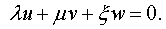The inverse relation is also interesting to note. The formula below is calculated in BarycentricsFormulas.html .
S denotes here the area of the triangle of reference ABC.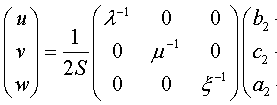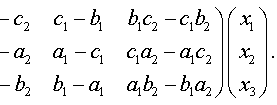4. Relation between two general homogeneous systems

The relation of homogeneous coordinates with respect to two systems {A,B,C,D} and {A',B',C',D'}
results from the previous by multiplying the appropriate matrices: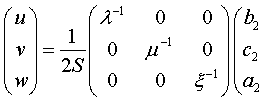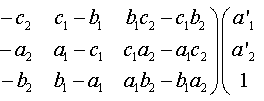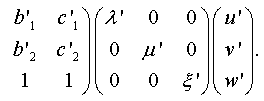In particular keeping fixed the triangle ABC and changing only D to D' gives for the corresponding
transition functions relating the two systems of homogeneous coordinates the simple rule: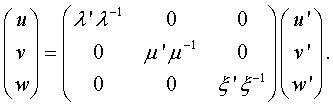5. Closer view of natural homogeneous coordinate system

This is the system resulting from the cartesian coordinates (x,y) of the plane by replacing x->x/z, y->y/z and adopting for
coordinates of the point the tripple (x,y,z). It is a projective system in which the line at infinity is z=0.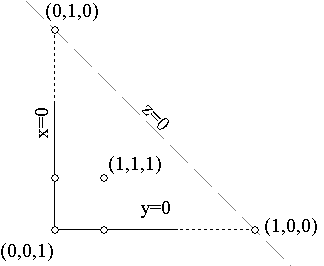This system can be considered as one example of those described in section-2 for which the triangle of reference has
one side identical with the line at infinity (z=0). Some of the assertions for general systems are not valid in this case.
For example the coordinates of the unit are (1,1,1) but these are not the barycentric coordinates with respect to the triangle
of reference, since this is infinite.

Remark-1 We obtain equations in other coordinate systems by homogenizing the known equations in
cartesian systems and transforming by the transformation rule of section-3. Thus, the line represented in cartesian
coordinates by an equation of the form ax+by+c=0 goes over to homoneous ax+by+cz=0, which transforming the
coordinates by an invertible matrix (x,y,z)t = M(x',y',z') obviously transforms in an equation of similar form
a'x'+b'y'+c'z'=0. Analogously is shown that a quadratic equation transforms to a quadratic equation etc..

Remark-2 The previous remark can be applied to find the formal equation of the radical axis of two circles
whose equations are x2+y2 + (ax+by+c) =0 and x2+y2+(a'x+b'y+c')=0. Homogenizing the equations and substructing
we obtain  z((a-a')x+(b-b')y+(c-c')z)=0. This represents the product of two lines: the line at infinity (z=0) and the
radical axis in homogeneous coordinates ( (a-a')x+(b-b')y+(c-c')z=0). By transforming to a general homogeneous system
the expression x2+y2 goes over to a standard quadratic one Q0 in terms of the new coordinates (x',y',z') and the remaining
terms transform to a product of lines L(x',y',z')L0(x',y',z') of which the second L0=0 is the line at infinity.
Thus, in the general coordinate system, in which the equation of the circle is written as a sum Q0(x,y,z)+L(x,y,z)L0(x,y,z)=0
in which Q0(x,y,z) is a standard expression (independent of the particular circle) and L0(x,y,z) is the line at infinity,
the difference L-L'=0 of the linear parts of the equations of two circles represents the radical axis of these circles.
See Remark-1 in section-2 of BarycentricsFormulas.html [LoneyII, p. 69].6. Rules of calculation

Using the projective systems of coordinates one can define operations of numbers on points of the
projective plane. The basic rule is that point X and point rX are the same. If (x,y,z) are the (homogeneous)
coordinates of X with respect to some fixed system, then rX can be considered to have coordinates
(rx,ry,rz) which define the same point. Every point of the plane can be represented as:
(1)                                                 X = uA + vB + wC.
Every point of the line defined by points {X,Y} can be written in the form:
(2)                                                 Pt = (1-t)X + tY.
An equation of the form  pX + qY = p'X + q'Y denotes a point Z which is common to the two lines
defined by {X,Y} and {X',Y'}: Z = pX + qY = p'X + q'Y'.
In a combination  Y = t1X1+t2X2 + ... all {ti} can be multiplied by the same number t and
the resulting point (tt1)X1 + (tt2)X2+... will represent the same point Y.
If points {X1, X2, X3} are not collinear i.e. they do not satisfy a relation of the form:
(3)                                                 t1X1 + t2X2 + t3X3 = 0,
then for every other point X there are three numbers, defined up to multiplicative constant, such that:
(4)                                                 X = t1X1 + t2X2 + t3X3.
Often using such representations of points and these rules of computation one can give simple proofs
of seemingly complicated theorems. As an example let us prove Desargue's theorem (see Desargues.html ):

Theorem If two triangles ABC, A'B'C' of the same plane are point-perspective then
they are also line-perspective.

Point-perspectivity means that there are lines between corresponding vertices passing through the
same point D. Line-perspectivity means that there are pairs of corresponding sides intersecting
at three collinear points on a line d.
Assume now that ABC, A'B'C' are point-perspective and there is a point D such that:
(i) D = aA+a'A' (is on line AA'), (ii) D = bB+b'B' (is also on BB'), (iii) D = cC+c'C' (is also on CC').
Thus aA + a'A' = bB+b'B' => aA-bB = -a'A'+b'B = X is a point on both lines AB and A'B' hence it is
their intersection. Analogously bB-cC = -b'B'+c'C' = Y is the intersection of BC and B'C' and
cC-aA = -c'C+a'A' = Z is the intersection of CA and C'A'. But X+Y+Z = (aA-bB)+(bB-cC)+(cC-aA)=0
implies that the three points are collinear.7. Cross ratio, harmonicity

Using the previous notation and denoting four points of the line XY by  A=X+aY, B=X+bY, C=X+cY, D=X+dY
their cross ratio is the number (see ProjectiveBase.html ):
(5)                                  (A,B,C,D) = [(a-c)/(b-c)]:[(a-d)/(b-d)].
Four points are said to form a harmonic division when (A,B,C,D)=-1.
Given three points {A,B,C} the harmonic fourth or harmonic conjugate of C with respect
to {A,B} is a point D, denoted some times with D=(A,B)/C and satisfying  (A,B,C,D)=-1. This relation of
harmonicity is handled in  section-3 of ProjectiveBase.html , where it is seen that the harmonic conjugate
of Z = X+zY with respect to {X,Y} is Z'=(X,Y)/Z = X-zY.
An interesting construct related to cross-ratio and harmonicity is the trilinear polar of a point with
respect to a triangle, handled also in the aforementioned file.8. Tripole of line at infinity

As an exercise in homogeneous coordinates let as calculate the tripole of the line at infinity represented
by (0). First the intersection points of the axes {u=0, v=0, w=0} with this line are correspondingly points: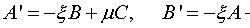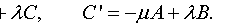According to the previous section the harmonic conjugates {A''=(B,C)/A', B''=(C,A)/B', C''=(A,B)/C'} are: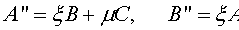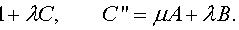Lines {AA'', BB'', CC''} intersect at a point E, which can be located by solving the following equations
with respect to {x,y,z}: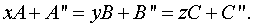For example the first equation is equivalent to:
xA + A'' = k(yB+B''),
since multiplication by arbitrary constants is allowed. Replacing in this the values for A'', B'' gives: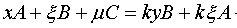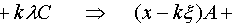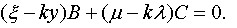By the independence of {A,B,C} this implies the vanishing of the coefficients, hence: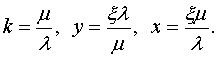Introducing this into the expression for the common point E = xA+A'' we obtain: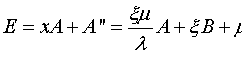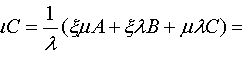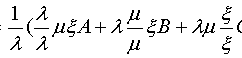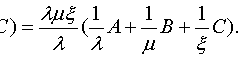This,  by the invariance for multiples with scalars, is equivalent to (see Trilinears.html , section ):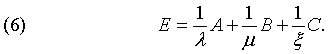9. Curves in homogeneous coordinates

Lines are expressed in homogeneous coordinates (x,y,z) through linear equations of the form:
(5)                                                                 ax + by + cz = 0.
They can be also described in parametric form using the projective basis of the system (A,B,C):
(6)                                                Xt = (a1t+a0)A + (b1t+b0)B + (c1t+c0)C.
Higher degree curves such as conics, cubics, quartics, quintics etc.. are of interest in projective geometry.
Analogously to (5) they are defined by homogeneous functions of the three homogeneous coordinates
(x,y,z) through equations such as:
(7)                                               ax2+2hxy+by2+2gxz+2fyz+cz2 = 0   (conics).
(8)                                   ax3+by3+cz3+dx2y+ey2z+fz2x + gx2z+hy2x+kz2y+mxyz = 0 (cubics).
Some properties of the conics expressed through homogeneous coordinates are discussed in
Conics_In_Homogeneous.html .

ProjectiveBase.html
BarycentricCoordinates.html
Trilinears.html
BarycentricsFormulas.html
Desargues.html
Conics_In_Homogeneous.html

Bibliography

[LoneyII] Loney, S. L. Coordinate Geometry, vol. II Trilinear Coordinates Macmillan, London 1934